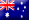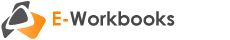# Accounting for Decision MakingIntroduces the contents of financial statements and their interpretation and analysis. Introductory management accounting topics are also included. Emphasis is placed on covering the decision making tools from a user's perspective, without the need to refer to debits and credits.

## Part 1: Financial accounting

#### Introduction to accounting

• The role of accounting
• Users of accounting information
• Regulatory environment

• Partnerships
• Companies
• Financial statements of each business structure

#### Introduction to financial statements

• Defining assets, liabilities, equity, income, expenses and profit
• Classifying accounts as assets, liabilities, equity, income or expenses
• Assets = Liabilities + Equity
• Characteristics of financial statements
• Introduction to the balance sheet
• Introduction to the income statement

#### Transaction analysis

• The principle of duality
• Recording transactions to start up a business
• Recording income and expense transactions
• Recording transactions that affect inventory
• Multiple transaction analysis

#### Balance sheet

• The definition and recognition criteria of assets, liabilities and equity
• Applying the definition and recognition criteria
• Current and non-current classifications
• Transactions affecting the balance sheet
• Format, presentation and limitations of the balance sheet

#### Income statement

• The definition and recognition criteria of income and expenses
• Applying the definition and recognition criteria
• Cash vs accrual accounting
• Transactions affecting the income statement
• Determining gross and net profit
• Format and presentation of the income statement

#### Cash flow statement

• Purpose and format of the cash flow statement
• Operating, investing and financing cash flows
• Direct method of preparing the cash flow statement
• Indirect method of preparing the cash flow statement
• Interpretation and analysis of cash flows

#### Financial statement analysis

• Horizontal and vertical analysis
• Calculating ratios
• Interpreting ratios
• Using financial statement analysis in decision making

Ratios covered include:

• Current ratio
• Quick ratio
• Cash flow from operations ratio
• Return on equity
• Return on assets
• Net profit margin
• Gross profit margin
• Interest cover / times interest earned
• Debt to equity ratio
• Debt ratio
• EPS
• P/E
• Asset turnover
• Average inventory turnover period/Inventory turnover (days)
• Average debtors settlement (debtors turnover (days))

## Part 2: Management accounting

#### Costing

• Direct and indirect costs
• Product and period costs
• Job costing and process costing
• Allocating overhead to a single product or service
• Determining costs in multi-product (multi-service) firms
• Using cost information to determine selling price and profit
• Using cost information to determine the value of inventory

#### CVP analysis

• Cost behaviour: variable, fixed and mixed
• CVP graph
• Break-even analysis
• Target profit and CVP analysis
• Using CVP analysis in decision making

#### Budgeting

• The budgeting process and the master budget
• Preparation of the sales, production and purchases budgets
• Preparation of the cash budget
• Preparation of the budgeted financial statements
• Flexible budgets
• Budget variance analysis

#### Capital investment decisions

• The nature of capital investment decisions
• Time value of money
• Accounting rate of return/Return on average investment
• Payback period
• Net present value
• Internal rate of return
• Using capital investment techniques in decision making, including multiple and mutually exclusive projects

#### Relevant costs and decision making

• Relevant costs and opportunity costs
• Incremental/differential analysis
• Special pricing/special order decisions